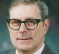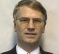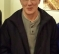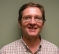## Digital IIR Parallel ImplementationStarted by 2 years ago15 replieslatest reply 2 years ago211 views

Hi All,

If i want to implement a simple IIR in digital design  y(n) = x(n) + a*y(n-1) with following constraint.

1. The input rate 6MHz that and y(n) should be maintained at the same rate

2 The multiplier though works only @ max 3 MHz

You can use more than one multiplier .

In a FIR setup such thing can be easily parallelised using even/odd inputs but how can the IIR be implemented parallley ?

[ - ]Write the z-transform of the transfer function.

H(z) = 1/(1-az^{-1})

Multiply the transfer function by (1+rz^{-1})/(1+rz^{-1})  which is 1. Choose r=-a.

Now the denominator has become (1-a^2 z^{-2}) so your difference equation will depend only on y(n-2) and you have time to complete the multiplication.  But just barely!!! You have put a zero at -a and a cancelling pole at -a.

A less flaky step would be to put two extra poles in numerator and the same poles in denominator. The poles would be at -r and -s and so the denominator would become

1 + (r+s-a) z^{-1} + (rs-(r+s)a) z^{-2} -ars z^{-3}.

Now choose r and s so that rs = a^2; rs=(r+s)a; r+s=a). Now you have a numerator with two multiplications and a denominator with only one multiplication by a^3. The filter output at sample n now depends only on y(n-3).  So your multiplier would have to be able to run at 2 MHz.

For a parallel implementation, you have separate realizations for, in the first case, odd numbered and even numbered sample outputs, and in the second case, for outputs 3n, 3n+1, 3n+2. In each of these cases, there are extra multiplications in the numerator but they can be done in parallel with no problem.

It has been many decades since I first saw this trick, which was invented by Ben Gold if I remember correctly.

One caution  -  to get good cancellation between the poles and zeroes the numerator polynomial roots must not be too sensitive to small variations in the numerator coefficients. One way to make sure of that is to use two (or three) numerator filters in series, e.g. (1-a z^{-1}) then (1- r z^{-1}) then (1-s z^{-1}).

[ - ]Oh man -- I've been trying to figure out how to do this for years.  And this is so obvious, and extensible to just about any first or second-order system.

I would feel shame at being so obtuse, but I'm too busy being happy that someone has skinned this cat for me.

You'd have to be careful that your nifty IIR solution actually remains smaller than an FIR -- the filter is certainly getting complex, and each extra delay you have to add means another set of multipliers (at least -- assuming no pipelining).

[ - ]You are exactly right. I said that in my first reply, in which I showed how to get away with  a 2:1 speed reduction, then suggested that you could use the same trick again to get a second 2:1 reduction. But that leaves you with a high order polynomial in the numerator, which means a lot of multiplies per sample. In the limit or many such reductions, you have

y(n) = sum_n a^m x(n-m) summed to infinity.

but I edited that out when I revised it to show the case of dependence on  y(n-3), which is closer to sudarshan_onkar's actual stated question.

[ - ]Wow !! This is a really powerful technique and it would have taken me few days to even think on these lines.  Thanks a million !!

[ - ]try avoid such slow mult e.g. using power of 2 or table.

[ - ]You can't really do a parallel system because each output feeds into the next input.  But you can build a pipeline so that each clock does multiple things.  Expand y(n) back a few terms, and then look at that many terms in the future.  Use the common multiplies to form each term.  So you will have a delay from input to output based on the depth of the pipeline.

This sounds like a homework problem.  In real life you just get a faster processor.

[ - ]Hi drmike,

This is something I thought about but pipelining is good alternative. I will think on these lines to pursue this.

Oh no this is a real problem i am facing in my work.

[ - ]Do make sure that if x[0...n] = 1, that you have stability, rather than an exponential 'explosion'.

That is, don't forget the effective multiplier for the x[n] term if the average of the x[n] terms is non-zero. e.g. (1-a) for your simple IIR design

[ - ]Philip-

Often such a lowpass is written:

y(n) = a*x(n) + b*y(n-1)

where a + b = 1 to maintain stability. Looks to me like Charlie's method could still be used, and input could be arbitrary.

-Jeff

[ - ]The concept of speed is completely different between hard dsp(ASIC/FPGA) and soft DSP.

Being an FPGA designer I relate speed directly to the [combinatorial-registers-combinatorial] chain.

The FPGA by nature needs registers for synchronous transfer of combinatorial decisions. The IIR delay stage serves that purpose as well. Here in this example we have adder(combinatorial) followed by multiplier(combinatorial) followed by a register. The only way to shorten the combinatorial loop is insert another register between adder and multiplier but the filter changes to y(n) = x(n) + y(n-2). If that is ok so be it.

For soft DSP speed is about fetch-execute time and the processing unit speed. I leave that to people in the field.

[ - ]Sudarshan_onkar,

On of the minor realities we often forget to teach undergraduate students is that the FIR filter can operate at rates that exceed the multiplier rate but that the canonic IIR filter rate is bounded by the speed of its multipliers. In the IIR filter, we can not compute the next output until the previous output has been computed. parallelism doesn't help.

There is however a trick we can use in which we design the IIR filter to operate at a reduced output rate, by embedding a 2-path, 2-to-1 or a 4-path 4-to-1 down sampler in its structure. each path operates at the reduced rate of f_in/2 or f_in/4. We then follow the filter output with a 2-path 1-to-2 up sampler or a 4-path 1-to-4 up sampler filter. This of course requires the output bandwidth to satisfy Nyquist rate at the reduced intermediate sample rate. The recursive filters can be designed with non-uniform phase (no surprise) or with equal-ripple linear phase (big surprise). These filters interchange the filtering and sample rate change, so the sample rate change occurs on the way in to the down sampling filter and on the way out of the up-sampling filter. This is contrary to what we learned about linear time invariant filters. These filters are linear time varying filters and operate under a different set of rules! What a great find!

What you want to do is see chapter 11 of the fred harris text, "Multirate Signal processing for Communication Systems): In the mean time till you pick up a copy, here is a short paper comparing an M-path resampling FIR, an M-path non uniform phase IIR, and an M-path uniform phase IIR. Mind boggling!  The thing you need to use these filters is the design routines to compute the coefficients of each path as well as an understanding of the filter structure of the IIR paths. Look in the IEEE-xplor if you have access to it for all-pass M-path filters, under my name for a start to see pretty good introductory tutorials. Markku Renfors wrote two very papers in the IEEE proceedings on this are: my design algorithms are based on these two papers. Anthony Constantinides also wrote some early papers on M-path Al-pass filters.

best regards

fred h

sdr_2005_Paper_polyphase.pdf

[ - ]this is another short paper comparing three versions of resampling filters in cascade. I am limited in what I can upload by page size restrictions... sorry about that

look for paper on line

Comparing LTE Channelizers Implemented with

Linear Phase Recursive Filters and FIR Filters

fred harris, Chris Dick, Elettra Venosa and Xiaofei Chen

San Diego State University

Xilinx Corp.

fred.harris@sdsu.edu, chris.dick@xilinx.com

evenosa@projects.sdsu.edu, chenxiaofei_sdsu@yahoo.com

fred h

[ - ]Post deleted by author

[ - ]Is there a Matlab example of an upsampling 1:2 Hilbert transform shown in that paper?  I follow the downsampling Hilbert transform in chapter 6 of your book but don't understand how to implement the reverse.

Thank you much,

Mark Napier

[ - ]